# Selecting Critical Components: Components Related to Snubber Circuits

2019.01.10

・A snubber circuit is incorporated in order to suppress surges arising from the leakage inductance of a transformer at the input.

・A basic snubber circuit has an RCD configuration, but for more complete protection, TVS diodes can be added.

In this article, instead of components for setting functions of the BD7682FJ power supply IC, we explain the constituent components and component values for snubber circuits that are frequently used in power supply circuits. Use of snubber circuits is not limited to the quasi-resonant converters of this article, but are also used in flyback converters, examples of which are given in a separate chapter. In conjunction with this article, please also refer to “Designing Isolated Flyback Converter Circuits: Selecting Critical Components ?CIN and Snubber” and “Isolated Flyback Converter Basics: Flyback Converter Operation and Snubber”.

## What is Snubber Circuit?

A snubber circuit is a circuit to suppress surges. In this example, it is provided at the input in order to suppress input surges, but use at the output is also possible. Because the input is connected to the primary side of a transformer, the leakage inductance of the transformer results in the occurrence of large surge voltages (spike noise) at instants when a MOSFET switches from on to off. This surge voltage is applied across the drain and source of the MOSFET, and so if the surge voltage exceeds the MOSFET voltage rating, the MOSFET may fail. In order to prevent this, an RCD (resistor, capacitor, diode) snubber circuit is inserted to suppress surge voltages. Surges occur in nearly all cases, and it is therefore recommended that a snubber circuit be provided right from the start.

## Snubber Circuit： Rsnubber1, Csnubber1, D13, D14, D15 and D16

The snubber circuit used in this example is formed from a resistor Rsnubber1, a capacitor Csnubber1, and diodes D13, D14, D15, D16. If D15 and D16 are removed, we have a typical RCD snubber circuit. First we determine the clamp voltage and the clamp ripple voltage, and then determine the component values in the order R, C, D.

1) Clamp voltage （Vclamp）and clamp ripple voltage（Vripple）

The clamp voltage is determined on the basis of the MOSFET voltage rating, including a margin. The margin is set at 20%.

Vclamp＝1700V×0.8＝1360V

The clamp ripple voltage (Vripple) is set to about 50 V.

2）Resistor Rsnubber1

Rsnubber1 is set so as to satisfy the following condition.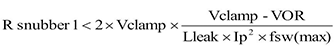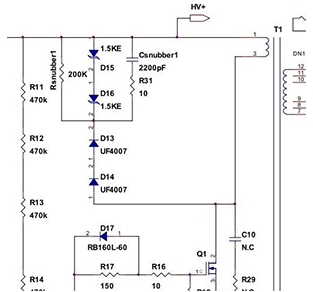Here the leakage inductance is set to Lleak=Lp×10%=1750 μH×10%=175 μH.

Using the following equations, Ip and fsw are calculated when Po=25 W and VIN(max)=900 V.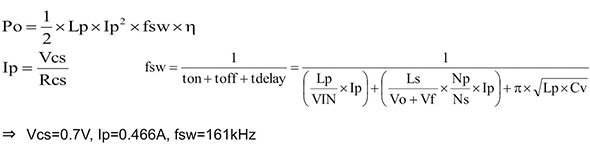From this we have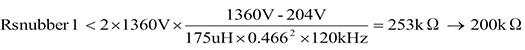The fsw value is adjusted from 161 kHz to 120 kHz because, as explained previously, the maximum switching frequency of the power supply IC is 120 kHz. Rsnubber1 should be a smaller value than the calculation result of 253 kΩ, and so is set to 200 kΩ.

The Rsnubber1 loss P_Rsnubber1 can be calculated as follows.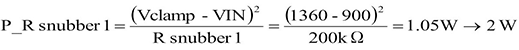With a margin included, a value of 2 W or greater is assumed. Consequently Rsnubber1 is set to 200 kΩ given the loss value of 2 W.

3）Csnubbe1
The electrostatic capacitance of Csnubber1 is calculated using the following equation.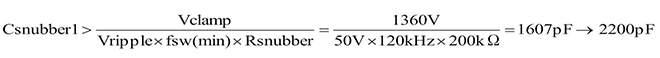As a capacitance larger than 1607 pF, we choose 2200 pF.

To determine the rated voltage of Csnubber1, because the voltage applied to Csnubber1 is the voltage Vclamp less VIN(max), or 1360 V-900 V=460 V, with a margin included, the rated voltage is set to at least 600 V. Here, then, we use an X7R 2200 pF, 2kV, 10% ceramic capacitor in a 1210 package.

4）D13、D14
Among the four diodes, fast recovery diodes are used for D13 and D14. The rated voltages are set to at least 1700 V, which is Vds(max) of the MOSFET. Here, we use two series-connected UF4007 diodes (1000 V, 1 A).

Surge voltages are affected not only by the transformer leakage inductance, but also by the parasitic components of thin film printed wiring, and so it is necessary to confirm the value of Vds while mounted on an actual board and then make adjustments to the snubber circuit according to the actual voltage.

5）D15、D16
These diodes are TVS (transient voltage suppressor) diodes, used for surge absorption. When more complete protective functions are demanded, TVS diodes can be added to clamp transient spike noise. The waveform during MOSFET switching is checked to determine whether such diodes should be used. The calculated voltage applied to these components is the same 460 V applied to Csnubber1, and so two 1.5KE200A diodes with a clamp voltage of 274 V are used in series, to clamp transient voltages higher than this.